Home

# Exact Hausdorff and packing measures of Cantor sets with overlaps

## Abstract

Let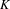$K$ be the attractor of a linear iterated function system (IFS)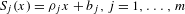$S_{j}(x)={\it\rho}_{j}x+b_{j},j=1,\ldots ,m$ , on the real line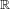$\mathbb{R}$ satisfying the generalized finite type condition (whose invariant open set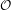${\mathcal{O}}$ is an interval) with an irreducible weighted incidence matrix. This condition was recently introduced by Lau and Ngai [A generalized finite type condition for iterated function systems. Adv. Math.208 (2007), 647–671] as a natural generalization of the open set condition, allowing us to include many important overlapping cases. They showed that the Hausdorff and packing dimensions of$K$ coincide and can be calculated in terms of the spectral radius of the weighted incidence matrix. Let${\it\alpha}$ be the dimension of$K$ . In this paper, we state that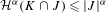$$\begin{eqnarray}{\mathcal{H}}^{{\it\alpha}}(K\cap J)\leq |J|^{{\it\alpha}}\end{eqnarray}$$
for all intervals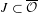$J\subset \overline{{\mathcal{O}}}$ , and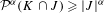$$\begin{eqnarray}{\mathcal{P}}^{{\it\alpha}}(K\cap J)\geq |J|^{{\it\alpha}}\end{eqnarray}$$
for all intervals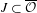$J\subset \overline{{\mathcal{O}}}$ centered in$K$ , where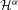${\mathcal{H}}^{{\it\alpha}}$ denotes the${\it\alpha}$ -dimensional Hausdorff measure and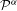${\mathcal{P}}^{{\it\alpha}}$ denotes the${\it\alpha}$ -dimensional packing measure. This result extends a recent work of Olsen [Density theorems for Hausdorff and packing measures of self-similar sets. Aequationes Math.75 (2008), 208–225] where the open set condition is required. We use these inequalities to obtain some precise density theorems for the Hausdorff and packing measures of$K$ . Moreover, using these density theorems, we describe a scheme for computing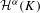${\mathcal{H}}^{{\it\alpha}}(K)$ exactly as the minimum of a finite set of elementary functions of the parameters of the IFS. We also obtain an exact algorithm for computing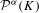${\mathcal{P}}^{{\it\alpha}}(K)$ as the maximum of another finite set of elementary functions of the parameters of the IFS. These results extend previous ones by Ayer and Strichartz [Exact Hausdorff measure and intervals of maximum density for Cantor sets. Trans. Amer. Math. Soc.351 (1999), 3725–3741] and by Feng [Exact packing measure of Cantor sets. Math. Natchr.248–249 (2003), 102–109], respectively, and apply to some new classes allowing us to include Cantor sets in$\mathbb{R}$ with overlaps.

Hide All

# Exact Hausdorff and packing measures of Cantor sets with overlaps

## Metrics

### Full text viewsFull text views reflects the number of PDF downloads, PDFs sent to Google Drive, Dropbox and Kindle and HTML full text views.

Total number of HTML views: 0
Total number of PDF views: 0 *Loading metrics...

### Abstract viewsAbstract views reflect the number of visits to the article landing page.

Total abstract views: 0 *Loading metrics...

* Views captured on Cambridge Core between <date>. This data will be updated every 24 hours.

Usage data cannot currently be displayed